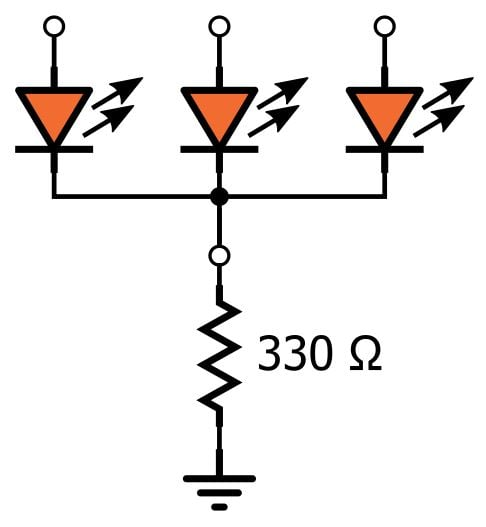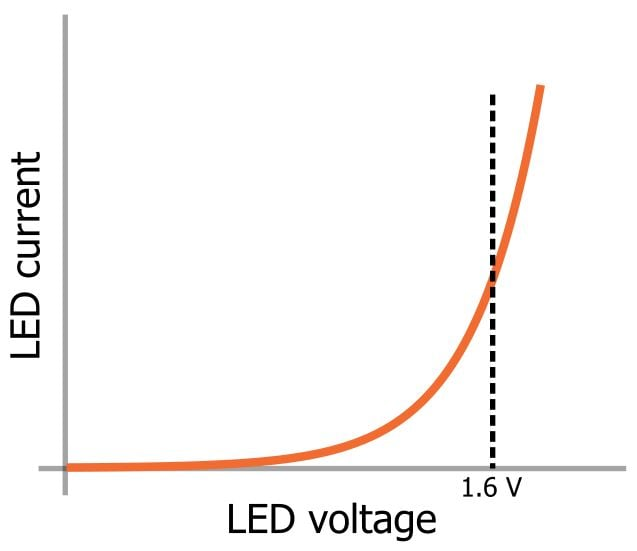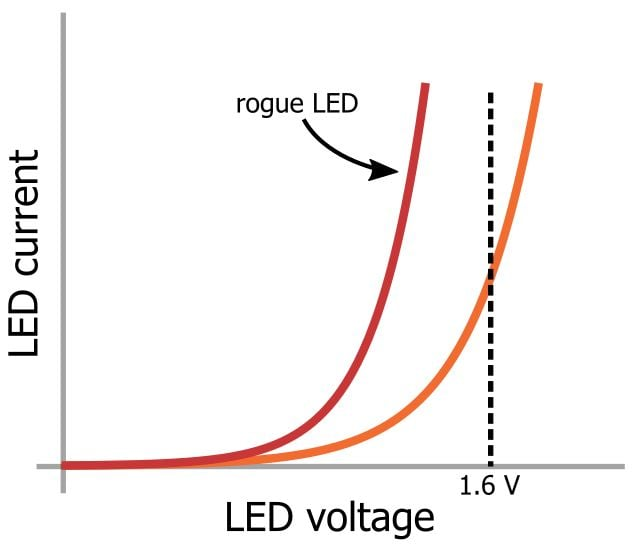Technical Article

# LED Arrays: One Resistor or Many?

November 15, 2016 by Robert Keim

## When you have multiple LEDs with a common anode or common cathode, can you use just one current-limiting resistor? Let’s take a look.

When you have multiple LEDs with a common anode or common cathode, can you use just one current-limiting resistor? Let’s take a look.

### Related Information

One of the most common, if less than thrilling, aspects of electronics design is choosing the right amount of resistance for limiting the current through the ubiquitous little indicators known as LEDs. The procedure is not particularly complicated—we assume a constant voltage drop across the LED and then do a little math to determine the resistance that will give us the desired forward current.

The constant-voltage assumption is completely inconsistent with reality, but we get away with it because generally we don’t mind if the LED current is a little higher or lower than expected.

But the constant-voltage assumption fails us when we are dealing with an array of LEDs integrated into a single package—for example, a seven-segment display. It fails us because it leads to a conundrum: If we assume a constant (and equal) voltage drop for all the LEDs in the device, we can drive the entire display with only one current-limiting resistor. And yet, it seems that everyone decides to use separate resistors for each LED.

Consider the following circuit, which represents a device that has three common-cathode LEDs in one package.Let’s assume that the forward voltage (VF) for each LED is 1.6 V. If we apply a 5 V drive signal to each pin, the common-cathode voltage is 3.4 V, and then the current through the resistor is 10.3 mA. Because each LED has the same voltage drop, we assume that they have the same current, so the forward current (IF) for each LED is 3.4 mA. Done deal—why bother with three resistors?

There are two issues here: First, the voltage drop is not constant. Second, we cannot assume that the three LEDs have exactly the same current–voltage characteristics.

The actual current through the LED is governed by an exponential relationship, such as the following:Note two things:

• VF can be considered approximately constant once the LED is in full conduction because even large increases in IF correspond to small changes in VF.
• In the region where the slope of the exponential curve is rapidly increasing, small changes in VF correspond to large changes in IF.

Now let’s imagine that one of the LEDs has a current–voltage characteristic that is shifted to the left relative to those of the other two.When voltage is applied, this troublesome LED will enter full conduction at, say, VF = 1.3 V and, because all the LEDs share a cathode, this one LED will limit the voltage across the other LEDs to 1.3 V. This is a problem because, for the other two LEDs, 1.3 V corresponds to only small amounts of current.

The point here is that you generally don’t want to use only one current-limiting resistor because you can’t ensure that the LEDs will equally share current; furthermore, it is possible that one LED will get much more current than the others.

However, LEDs contained within a single package should exhibit fairly consistent current–voltage characteristics (unless they are intentionally inconsistent, such as with an RGB LED module). Thus, a single current-limiting resistor might provide adequate performance in many applications—but remember to account for the power dissipation! Power is proportional to the square of current, and the current through the single resistor can become quite large if you need, say, eight LEDs all operating at significant brightness.

Apart from the inconveniently high power dissipation with the single-resistor approach, the bottom line is that individual resistors for each LED is the preferred way to drive an LED array.• S
softwaremonkey November 18, 2016

Good article but this is only relevant in static display drivers. It is OK (and very common) to use a single resistor with multiplexed display drivers.

Like.
• R
rfaass November 18, 2016

Not all led are on simultaneously…

Like.
• B
billbucket November 18, 2016

This is really very WRONG. You forgot to mention the most critical reason you wouldn’t do this with something like the seven segment display you use as an example. Not all the LEDs will be on at the same time. Depending on what character you’re displaying, the current through a single resistor will change. If you calculate the resistor for all seven LEDs being on then only turn on two (for a 1 digit) you will be driving too much current through those LEDs. You should really post the *correct* schematic, rather than the version that will cause the circuit you’ve described to fail.

Like.
•jkwilborn November 20, 2016
I was about to point this out... As with the engineering, you are limiting the current to each segment by a resistor. To do that you need a current limiting resistor on EACH segment. This article should have started with "why you don't..."
Like.
•N11778 November 22, 2016
• B
billbucket November 18, 2016

A single limiting resistor for an RGB will not work either! The different colors of LEDs typically have very different forward voltage drops and, again, you can only calculate the current for certain combinations of the LEDs. If you use the resistor that would satisfy current limiting for all three LEDs and turn only one on you will likely damage your part! This is a very bad idea!

Like.